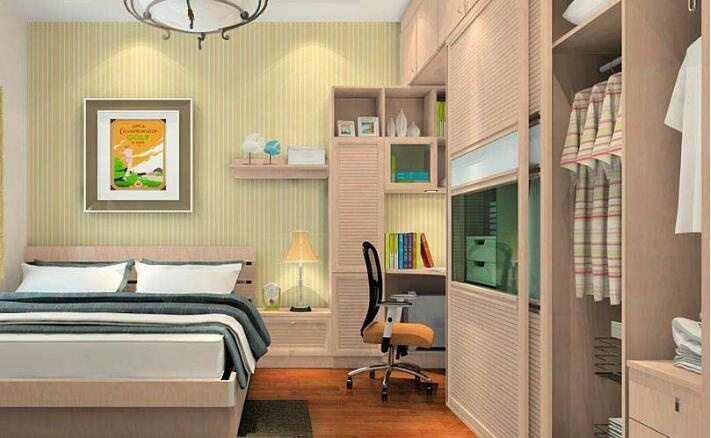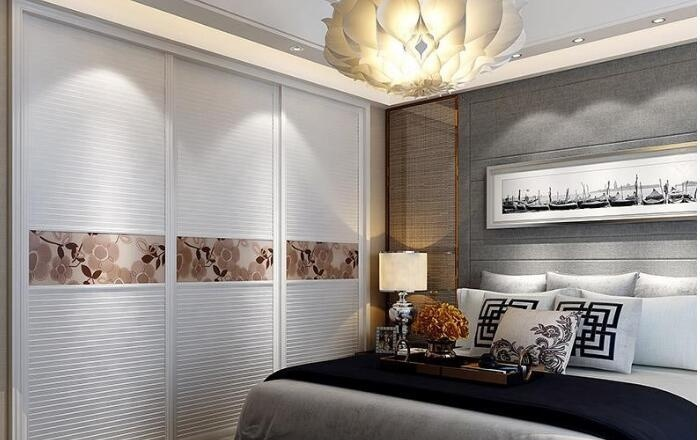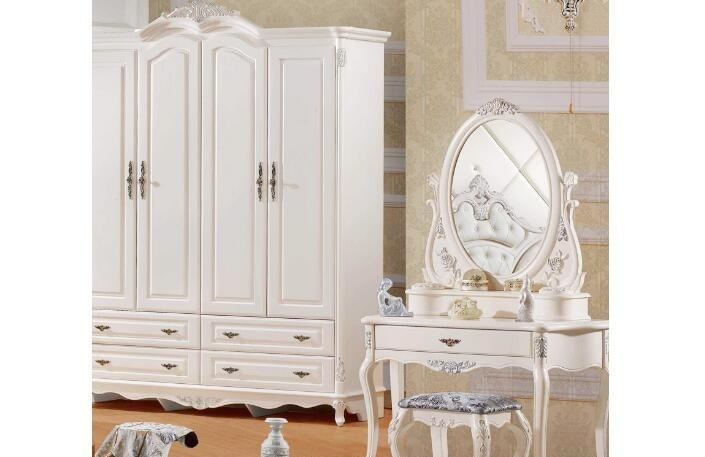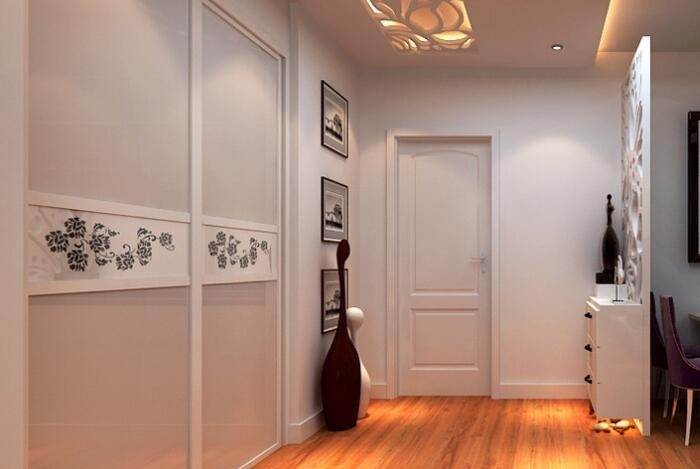|

# 中国十大整体衣柜 2016中国十大整体衣柜排名

整体衣柜经常放在我们的卧室中，我们在选购整体衣柜的时候都想选购一款材质比较环保，品牌比较知名的，市场上整体衣柜品牌是有很多的，我们在选购的时候经常会不知所措，那么中国十大整体衣柜排名怎么样呢，中国十大整体衣柜哪些比较好呢，下面我们就一起来了解一下吧。中国十大整体衣柜 2016中国十大整体衣柜排名

中国十大整体衣柜之：瑞丽宜家

瑞丽宜家，国内全屋定制家居行业的领军品牌。瑞丽宜家历经十年行业沉淀，融中西家居文化之精髓于一身，以国际化的标准和眼光，将高科技与时尚美学相融合，凭借一流的设计研发团队的创意力量和对消费流行趋势的敏锐把握，引领中国时尚家居风潮。

中国十大整体衣柜之：德维尔Deweier

德维尔家具有限公司，集设计、研发、生产、销售于一体，是中国优秀的定制家具企业。2005年，德维尔正式踏入定制家具行业，率先通过了ISO9001国际质量管理体系认证，并在有着“工业强市”之称的从化明珠工业园建设专属生产基地。随后，营销中心迁至广州天河华景新城，毗邻天河商圈，环境优美，交通便利，拥有全智能的、舒适的办公条件。中国十大整体衣柜之：梵帝尼Fantine

法国“Fantine梵帝尼”始创于1976年，在世界各地拥有20多家大型加工企业，制造销售高档家具、皮具、饰品、服装四大系列共二千多个品种，是欧美人家居生活理想之选。董事局主席Stéphane博士出生于法国没落的贵族家庭，自沃顿商学院毕业后，承接经营家族企业，将“Fantine梵帝尼”品牌由一个默默无闻的皮具品牌发展成为世界顶级品牌，“BestLifeStyle，BestFantine!”已渗入到每一个欧美人心目中。

中国十大整体衣柜之：玛格

创立以2004年的玛格集设计、制造、生产、销售整体衣柜于一身，秉着自主研发，合理经营的理念，迅速在国内发展壮大，成为无限非标定制的践行者。

中国十大整体衣柜之：诺维家

整体衣柜十大品牌排名之一，源自于意大利风格的家具品牌，其整体衣柜不论是时尚的现代风格，或是庄重的古典风格，都受到人们的喜爱。中国十大整体衣柜之：雅迪斯

雅迪斯整体衣柜是广州市恒雅家具实业有限公司旗下的品牌，该品牌风格多变、尺寸合理、个性突出、功能齐全、健康环保的优势使之在整体衣柜行业拥有一席之地。

中国十大整体衣柜之：诗尼曼

广州市诗意家具有限公司成立于2003年，其设计生产的诗尼曼衣柜是中国定制衣柜的创始品牌之一。诗尼曼以衣柜为中心，产品辐射定制衣柜、衣帽间、时尚书柜、电视柜、酒柜、高箱床、床头柜、装饰柜、梳妆台、鞋柜、隔断门、厅房组合柜，使消费者充分体验到定制家居的便捷性和个性化。

中国十大整体衣柜之：卡喏亚

广州市华标家具材料有限公司是集研发、设计、生产、销售、服务于一体的卡喏亚（Knoya）品牌衣柜及配套家具专营企业。卡喏亚品牌创立于2001年3月，作为中国最早的定制衣柜品牌。卡喏亚品牌产品主要包括定制衣柜、书柜、衣帽间、推拉门、配套家具、第三代组合家具等。所有产品均已达到欧洲E1级或更高的环保标准，并且采用激光标识防伪，让顾客买得放心，用得舒心。中国十大整体衣柜之：罗兰

罗兰是全球整体卧室的缔造者，罗兰不但颠覆式地创造了一个全新品类，同时也创造了一片蓝海，一个充满想象空间的巨大市场，罗兰整体卧室将深刻地影响中国整体家居的格局和未来，引领消费者从未拥有的整体卧室体验。由于罗兰在整体卧室的卓越成就和影响力，成就了整体卧室第一品牌。作为整体卧室的创导者，罗兰品牌基因与中国文化唇齿相依，中国市场已经成为罗兰全球战略的重要组成部分，充满生活美学的罗兰整体卧室已经成为“简约、时尚、优雅”生活方式的象征。

中国十大整体衣柜之：尚品宅配

尚品宅配成立于2004年，是广州尚品宅配家居用品有限公司旗下品牌，是一家强调依托高科技创新性迅速发展的家具企业。尚品宅配整体衣柜设计注重美观与实用的结合，直通到顶的柜体，收纳性极强，让每一寸空间都能用上，开敞式的整体衣柜可以清晰看到，个性化的收纳方式更可体贴到每一个小细节。

`声明：本文由入驻焦点开放平台的作者撰写，除焦点官方账号外，观点仅代表作者本人，不代表焦点立场错误信息举报电话： 400-099-0099，邮箱：jubao@vip.sohu.com，或点此进行意见反馈，或点此进行举报投诉。`A B C D E F G H J K L M N P Q R S T W X Y Z
A - B - C - D - E
• A
• 鞍山
• 安庆
• 安阳
• 安顺
• 安康
• 澳门
• B
• 北京
• 保定
• 包头
• 巴彦淖尔
• 本溪
• 蚌埠
• 亳州
• 滨州
• 北海
• 百色
• 巴中
• 毕节
• 保山
• 宝鸡
• 白银
• 巴州
• C
• 承德
• 沧州
• 长治
• 赤峰
• 朝阳
• 长春
• 常州
• 滁州
• 池州
• 长沙
• 常德
• 郴州
• 潮州
• 崇左
• 重庆
• 成都
• 楚雄
• 昌都
• 慈溪
• 常熟
• D
• 大同
• 大连
• 丹东
• 大庆
• 东营
• 德州
• 东莞
• 德阳
• 达州
• 大理
• 德宏
• 定西
• 儋州
• 东平
• E
• 鄂尔多斯
• 鄂州
• 恩施
F - G - H - I - J
• F
• 抚顺
• 阜新
• 阜阳
• 福州
• 抚州
• 佛山
• 防城港
• G
• 赣州
• 广州
• 桂林
• 贵港
• 广元
• 广安
• 贵阳
• 固原
• H
• 邯郸
• 衡水
• 呼和浩特
• 呼伦贝尔
• 葫芦岛
• 哈尔滨
• 黑河
• 淮安
• 杭州
• 湖州
• 合肥
• 淮南
• 淮北
• 黄山
• 菏泽
• 鹤壁
• 黄石
• 黄冈
• 衡阳
• 怀化
• 惠州
• 河源
• 贺州
• 河池
• 海口
• 红河
• 汉中
• 海东
• I
• J
• 晋中
• 锦州
• 吉林
• 鸡西
• 佳木斯
• 嘉兴
• 金华
• 景德镇
• 九江
• 吉安
• 济南
• 济宁
• 焦作
• 荆门
• 荆州
• 江门
• 揭阳
• 金昌
• 酒泉
• 嘉峪关
K - L - M - N - P
• K
• 开封
• 昆明
• 昆山
• L
• 廊坊
• 临汾
• 辽阳
• 连云港
• 丽水
• 六安
• 龙岩
• 莱芜
• 临沂
• 聊城
• 洛阳
• 漯河
• 娄底
• 柳州
• 来宾
• 泸州
• 乐山
• 六盘水
• 丽江
• 临沧
• 拉萨
• 林芝
• 兰州
• 陇南
• M
• 牡丹江
• 马鞍山
• 茂名
• 梅州
• 绵阳
• 眉山
• N
• 南京
• 南通
• 宁波
• 南平
• 宁德
• 南昌
• 南阳
• 南宁
• 内江
• 南充
• P
• 盘锦
• 莆田
• 平顶山
• 濮阳
• 攀枝花
• 普洱
• 平凉
Q - R - S - T - W
• Q
• 秦皇岛
• 齐齐哈尔
• 衢州
• 泉州
• 青岛
• 清远
• 钦州
• 黔南
• 曲靖
• 庆阳
• R
• 日照
• 日喀则
• S
• 石家庄
• 沈阳
• 双鸭山
• 绥化
• 上海
• 苏州
• 宿迁
• 绍兴
• 宿州
• 三明
• 上饶
• 三门峡
• 商丘
• 十堰
• 随州
• 邵阳
• 韶关
• 深圳
• 汕头
• 汕尾
• 三亚
• 三沙
• 遂宁
• 山南
• 商洛
• 石嘴山
• T
• 天津
• 唐山
• 太原
• 通辽
• 铁岭
• 泰州
• 台州
• 铜陵
• 泰安
• 铜仁
• 铜川
• 天水
• 天门
• W
• 乌海
• 乌兰察布
• 无锡
• 温州
• 芜湖
• 潍坊
• 威海
• 武汉
• 梧州
• 渭南
• 武威
• 吴忠
• 乌鲁木齐
X - Y - Z
• X
• 邢台
• 徐州
• 宣城
• 厦门
• 新乡
• 许昌
• 信阳
• 襄阳
• 孝感
• 咸宁
• 湘潭
• 湘西
• 西双版纳
• 西安
• 咸阳
• 西宁
• 仙桃
• 西昌
• Y
• 运城
• 营口
• 盐城
• 扬州
• 鹰潭
• 宜春
• 烟台
• 宜昌
• 岳阳
• 益阳
• 永州
• 阳江
• 云浮
• 玉林
• 宜宾
• 雅安
• 玉溪
• 延安
• 榆林
• 银川
• Z
• 张家口
• 镇江
• 舟山
• 漳州
• 淄博
• 枣庄
• 郑州
• 周口
• 驻马店
• 株洲
• 张家界
• 珠海
• 湛江
• 肇庆
• 中山
• 自贡
• 资阳
• 遵义
• 昭通
• 张掖
• 中卫

1室1厅1厨1卫1阳台

1
2
3
4
5

0
1
2

1

1

0
1
2
3报名成功，资料已提交审核A B C D E F G H J K L M N P Q R S T W X Y Z
A - B - C - D - E
• A
• 鞍山
• 安庆
• 安阳
• 安顺
• 安康
• 澳门
• B
• 北京
• 保定
• 包头
• 巴彦淖尔
• 本溪
• 蚌埠
• 亳州
• 滨州
• 北海
• 百色
• 巴中
• 毕节
• 保山
• 宝鸡
• 白银
• 巴州
• C
• 承德
• 沧州
• 长治
• 赤峰
• 朝阳
• 长春
• 常州
• 滁州
• 池州
• 长沙
• 常德
• 郴州
• 潮州
• 崇左
• 重庆
• 成都
• 楚雄
• 昌都
• 慈溪
• 常熟
• D
• 大同
• 大连
• 丹东
• 大庆
• 东营
• 德州
• 东莞
• 德阳
• 达州
• 大理
• 德宏
• 定西
• 儋州
• 东平
• E
• 鄂尔多斯
• 鄂州
• 恩施
F - G - H - I - J
• F
• 抚顺
• 阜新
• 阜阳
• 福州
• 抚州
• 佛山
• 防城港
• G
• 赣州
• 广州
• 桂林
• 贵港
• 广元
• 广安
• 贵阳
• 固原
• H
• 邯郸
• 衡水
• 呼和浩特
• 呼伦贝尔
• 葫芦岛
• 哈尔滨
• 黑河
• 淮安
• 杭州
• 湖州
• 合肥
• 淮南
• 淮北
• 黄山
• 菏泽
• 鹤壁
• 黄石
• 黄冈
• 衡阳
• 怀化
• 惠州
• 河源
• 贺州
• 河池
• 海口
• 红河
• 汉中
• 海东
• I
• J
• 晋中
• 锦州
• 吉林
• 鸡西
• 佳木斯
• 嘉兴
• 金华
• 景德镇
• 九江
• 吉安
• 济南
• 济宁
• 焦作
• 荆门
• 荆州
• 江门
• 揭阳
• 金昌
• 酒泉
• 嘉峪关
K - L - M - N - P
• K
• 开封
• 昆明
• 昆山
• L
• 廊坊
• 临汾
• 辽阳
• 连云港
• 丽水
• 六安
• 龙岩
• 莱芜
• 临沂
• 聊城
• 洛阳
• 漯河
• 娄底
• 柳州
• 来宾
• 泸州
• 乐山
• 六盘水
• 丽江
• 临沧
• 拉萨
• 林芝
• 兰州
• 陇南
• M
• 牡丹江
• 马鞍山
• 茂名
• 梅州
• 绵阳
• 眉山
• N
• 南京
• 南通
• 宁波
• 南平
• 宁德
• 南昌
• 南阳
• 南宁
• 内江
• 南充
• P
• 盘锦
• 莆田
• 平顶山
• 濮阳
• 攀枝花
• 普洱
• 平凉
Q - R - S - T - W
• Q
• 秦皇岛
• 齐齐哈尔
• 衢州
• 泉州
• 青岛
• 清远
• 钦州
• 黔南
• 曲靖
• 庆阳
• R
• 日照
• 日喀则
• S
• 石家庄
• 沈阳
• 双鸭山
• 绥化
• 上海
• 苏州
• 宿迁
• 绍兴
• 宿州
• 三明
• 上饶
• 三门峡
• 商丘
• 十堰
• 随州
• 邵阳
• 韶关
• 深圳
• 汕头
• 汕尾
• 三亚
• 三沙
• 遂宁
• 山南
• 商洛
• 石嘴山
• T
• 天津
• 唐山
• 太原
• 通辽
• 铁岭
• 泰州
• 台州
• 铜陵
• 泰安
• 铜仁
• 铜川
• 天水
• 天门
• W
• 乌海
• 乌兰察布
• 无锡
• 温州
• 芜湖
• 潍坊
• 威海
• 武汉
• 梧州
• 渭南
• 武威
• 吴忠
• 乌鲁木齐
X - Y - Z
• X
• 邢台
• 徐州
• 宣城
• 厦门
• 新乡
• 许昌
• 信阳
• 襄阳
• 孝感
• 咸宁
• 湘潭
• 湘西
• 西双版纳
• 西安
• 咸阳
• 西宁
• 仙桃
• 西昌
• Y
• 运城
• 营口
• 盐城
• 扬州
• 鹰潭
• 宜春
• 烟台
• 宜昌
• 岳阳
• 益阳
• 永州
• 阳江
• 云浮
• 玉林
• 宜宾
• 雅安
• 玉溪
• 延安
• 榆林
• 银川
• Z
• 张家口
• 镇江
• 舟山
• 漳州
• 淄博
• 枣庄
• 郑州
• 周口
• 驻马店
• 株洲
• 张家界
• 珠海
• 湛江
• 肇庆
• 中山
• 自贡
• 资阳
• 遵义
• 昭通
• 张掖
• 中卫• 手机• 分享
• 设计
免费设计
• 计算器
装修计算器
• 入驻
合作入驻
• 联系
联系我们
• 置顶
返回顶部